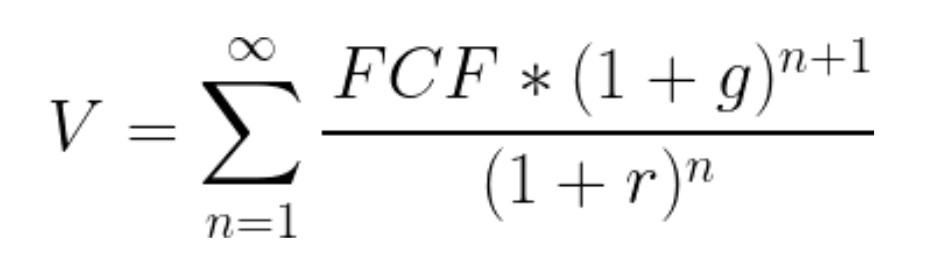Data processing in the USA: By clicking on "I accept", you also consent, in accordance with article 49 paragraph 1 sentence 1 lit. GDPR, to your data being processed in the USA (by Google LLC, Facebook Inc., LinkedIn Inc., Stripe, Paypal).

expert

Francesco

100% Recommendation Rate

3,414 Meetings

USD 449 / Coaching

6

# Why is NPV calculated as profit/discount rate

In this case, the NPV is calculated as profit/(discount rate-growth rate)-investment. Isn't NPV=FV/(1+i)^n?

In this case, the NPV is calculated as profit/(discount rate-growth rate)-investment. Isn't NPV=FV/(1+i)^n?

• Date ascending
• Date descending

Hi there,

The value generated by an asset in perpetuity can be calculated with the perpetuity formula as follows:

V=FCF/(r-g)

Where

V = Value of the asset

FCF = Free cash flow

r= Discount rate

g= Growth rate of FCF

Usually g=0 and FCF is approximated with profits for simplicity. If you want to calculate the NPV, you can then subtract the initial investment.

The previous formula for the value of an asset in perpetuity is equivalent to the following:Using the perpetuity formula allows you to calculate the value faster – which is why is normally used in case interviews instead of the second formula.

For a more technical explanation, you can look at the following link:

https://www.preplounge.com/en/consulting-forum/case-net-present-value-calculations-325

Best,

Francesco

Hi there,

The value generated by an asset in perpetuity can be calculated with the perpetuity formula as follows:

V=FCF/(r-g)

Where

V = Value of the asset

FCF = Free cash flow

r= Discount rate

g= Growth rate of FCF

Usually g=0 and FCF is approximated with profits for simplicity. If you want to calculate the NPV, you can then subtract the initial investment.

The previous formula for the value of an asset in perpetuity is equivalent to the following:Using the perpetuity formula allows you to calculate the value faster – which is why is normally used in case interviews instead of the second formula.

For a more technical explanation, you can look at the following link:

https://www.preplounge.com/en/consulting-forum/case-net-present-value-calculations-325

Best,

Francesco

For perpetuity (cash flows lasting forever), this formula is used: NPV = Profit/(discount rate-growth rate). The other formula is used when the time period is fixed.

For perpetuity (cash flows lasting forever), this formula is used: NPV = Profit/(discount rate-growth rate). The other formula is used when the time period is fixed.

Hi there,

I don't think the other coaches have really answered your question. You have 2 questions right?

1) You're asking why we subtract the investment?

Remember, NPV calculates today's value of future earnings. If we do NPV we get what all of our future profits are worth. Then, we need to subtract it from our upfront investment to make sure the NPV is larger than what we're paying today!

2) You're asking why we don't do "(1+i)^n"

Then, the reason we don't have "^n" is because that occurs when we have specific payouts in year 1, 2, etc. I.e. it does not go on forever. In the case above, we have cashflows into perpituity.

Hope this helps!

Hi there,

I don't think the other coaches have really answered your question. You have 2 questions right?

1) You're asking why we subtract the investment?

Remember, NPV calculates today's value of future earnings. If we do NPV we get what all of our future profits are worth. Then, we need to subtract it from our upfront investment to make sure the NPV is larger than what we're paying today!

2) You're asking why we don't do "(1+i)^n"

Then, the reason we don't have "^n" is because that occurs when we have specific payouts in year 1, 2, etc. I.e. it does not go on forever. In the case above, we have cashflows into perpituity.

Hope this helps!

Hello!

There are two ways to calculate NPVs:

• When we know the timeframe, then the formula you mention, NPV=FV/(1+i)^n, applies
• When we don´t and we are doing at perpetuity -meaning, all the cashflows annualized forever-, then we use the formula that is used in the problem

Hope it helps!

Cheers,

Clara

Hello!

There are two ways to calculate NPVs:

• When we know the timeframe, then the formula you mention, NPV=FV/(1+i)^n, applies
• When we don´t and we are doing at perpetuity -meaning, all the cashflows annualized forever-, then we use the formula that is used in the problem

Hope it helps!

Cheers,

Clara

Hey there!

It's quite simple - the time frame is not fixed, so the formula which you mention cannot be applied. Be careful with such conditions while case solving, it can change the outcomes dramatically.

If you have any other questions - write to me,

GB

Hey there!

It's quite simple - the time frame is not fixed, so the formula which you mention cannot be applied. Be careful with such conditions while case solving, it can change the outcomes dramatically.

If you have any other questions - write to me,

GB